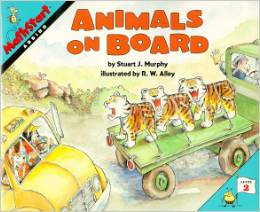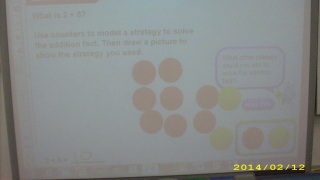# Basic Facts to 20

7 teachers like this lesson
Print Lesson

## Objective

SWBAT add and subtract facts within 20 and demonstrate fluency for addition and subtraction within 10.

#### Big Idea

As children extend their work with addition and subtraction strategies to basic facts to 20, they are building a foundation for later work with all numbers and operations.

## Activating Strategy

10 minutes

I begin this lesson by reading "Animals on Board"This book is contains simple addition problems, and has a sing-song rhythm to it.  I like to use it because it's a great refresher for any addition review, and it keeps the students engaged.

There is a You Tube version if the book isn't available.

I then place students in pairs and give each pair a domino.  Have the students count the dots on each side, use the two numbers as addends, and write two related addition facts.  Rotate students and dominos.

This opening activity gets the students thinking about math facts.  It encourages them to communicate precisely about their thinking because they have to work with a partner to write their math facts (MP6) ; and the dominoes provide them with a concrete structure that they then use to create a mathematical model (MP4).

As children extend their work with addition and subtraction strategies to basic facts to 20, they are building a foundation for later work with all numbers and operations. They will use strategies they have learned for basic facts to 10 to work out basic facts with numbers that now have two digits. They will see that they can use the same strategies when working with greater numbers.

It is important that children understand why a particular strategy works. For example, they can add 9 to a number by adding 10 and subtracting 1. For this strategy to be useful and extendable, children must understand that 9 is the same as 10 – 1. You can foster this understanding by asking children to explain why they chose a particular strategy and to describe what happens to the numbers when the strategy is used.

## Teaching Strategies

15 minutes

I write 5 + 4 = ___ on the board or chart paper (also available as a PPT Basic facts to 20)

I have students use two-color counters to model how to solve:  5 + 4 = _____

I guide the discussion to get them talking about the different strategies for addition:

• When you add two numbers, are you combining them, comparing them, or taking some away? (I am combining them.)
• How can you use two colors to show the problem? (I can use 5 counters of one color and 4 counters of the other color.)

I then write another addition problem on the board, and I ask a student to share their strategy for solving the problem.

I ask the students to use their counters to solve: 10 – 4 = ___.

Then I guide the discussion to get them talking about the different ways to solve subtraction problems:

• What subtraction fact do you need to find? (10 – 4)

I have a student to share their strategy for solving the subtraction problem, and the I continue with a few subtraction problems.

By sharing their strategies, students will develop a deeper understanding of the different ways to solve addition and subtraction problems.

Here is a video of the student demonstrating how he solved the problem 5 + 4:

This student chose to use counters to model how to solve the problem:## Independent Practice

30 minutes

For the independent practice portion of this lesson, students will solve various addition and subtraction problems to help develop their fluency and stamina, found on Basic facts to 20 worksheet.  Students should have access to counters, connecting cubes, or other manipulatives if they are needed.

For struggling students, have them use counters to model the problem:

• 5 people got on the bus.  Then 2 more people got on.  How many people are on the bus? (Have the students represent the problem with a number sentence after modeling with counters.)
• At the next stop, 3 people got off the bus.  How many people are left on the bus? (Have the student again represent the problem with a number sentence after solving with counters.)
• Continue the story until children understand the connections among the situations, the model, and the number sentence.

In this video, the student is using the strategy of counting on to solve a math problem.

## Closing/Summarizing

5 minutes

To close out this lesson, I like to give students an index card or post it with either an addition sentence, subtraction sentence or answer on the card/post it.  Students then circulate until they find the partner that has the card/post it that matches theirs.